# 第三次作业

1.设计思路

A，建立一个结构体，其中成员包括学号，姓名，成绩。

B，在主函数中，定义变量，然后输入学生人数，利用for循环依次输入学生学号，姓名，成绩。

C，再利用for循环求出学生成绩总和，然后求出平均成绩。

D，最后将低于平均成绩的学生姓名，学号输出。

2.流程图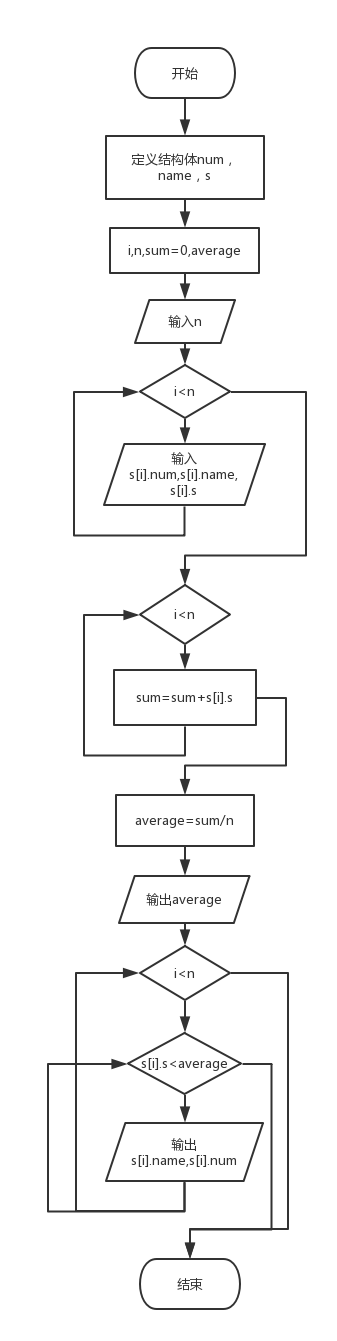3.实验代码

#include<stdio.h>
#include<string.h>
struct score
{
char num;
char name;
int score;
};
int main()
{
int i,n,count=0;
float average=0;
scanf("%d",&n);
struct score s[n];for(i=0;i<n;i++)
scanf("%s %s %d",&s[i].num,s[i].name,&s[i].score);
for(i=0;i<n;i++)
{
count+= s[i].score;
}
average = count / n;
printf("%.2f\n",average);
for(i = 0; i < n; i++)
{
if(s[i].score < average)
{
printf("%s %s\n",s[i].name,s[i].num);

}
}
return 0;
}

4.遇到的问题及解决方法：

pta提交总是部分正确，在dev上却可以运行，经过多次调试，后来在scanf("%d",&n);后加上一句struct score s[n];就好了。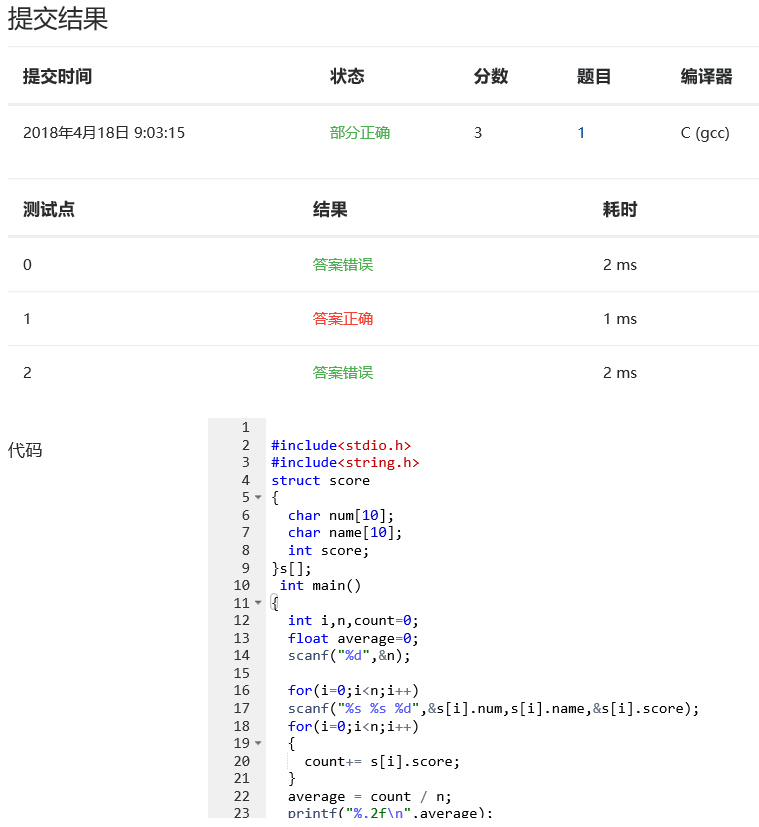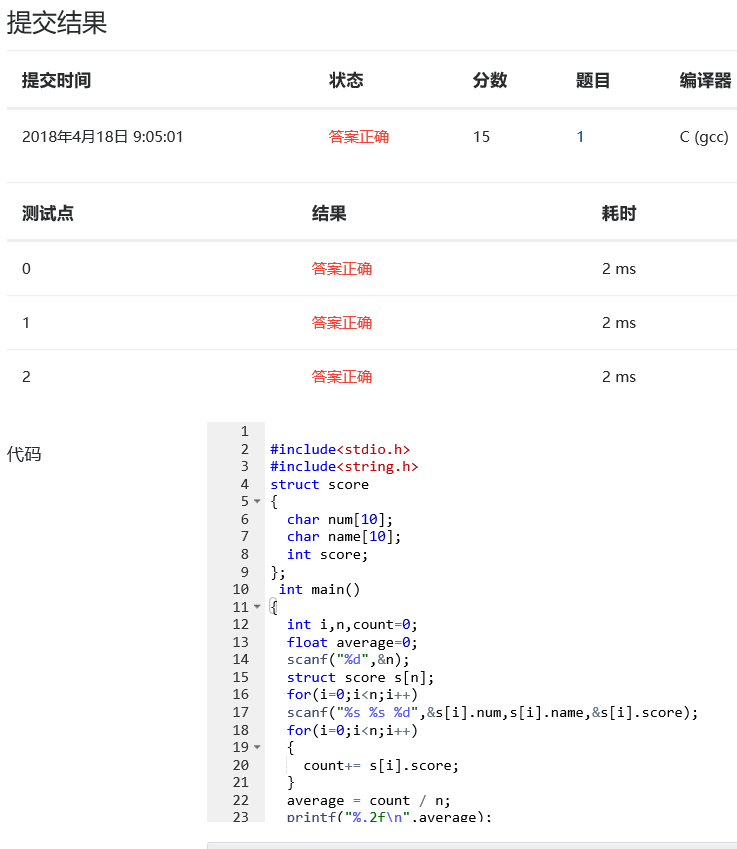1.设计思路

A，建立结构体，成员包括书名和价格。

B，在主函数中定义变量，输入需要查找的书籍数量，名字，价格。

C，利用循环结构找出书籍中的最高价和最低价，分别输出他们的名字和价格。

2.流程图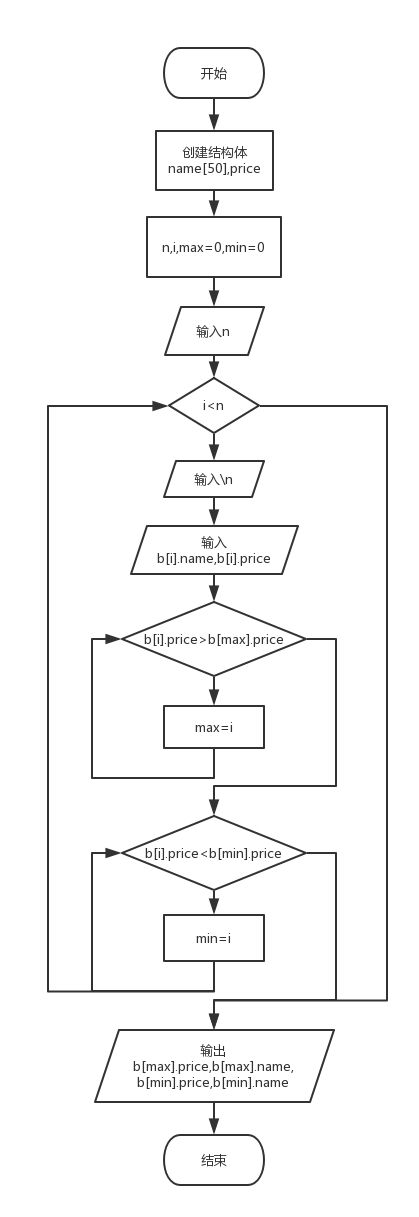3.实验代码

#include<stdio.h>
#include<string.h>
struct book{
char name;
double price;
}b;
int main(){
int n,i,j,mini=0,maxi=0;
double min=1000000,max=-1;
scanf("%d",&n);
for(i=0;i<n;i++){
scanf("\n");
gets(b[i].name);
scanf("%lf",&b[i].price);
if(b[i].price>max){
max=b[i].price;
maxi=i;
}
if(b[i].price<min){
min=b[i].price;
mini=i;
}
}
printf("%.2lf, %s\n",b[maxi].price,b[maxi].name);
printf("%.2lf, %s\n",b[mini].price,b[mini].name);
return 0;
}  

4.遇到的问题及解决方法：pta提交总是答案错误，在dev上却可以运行，多次调试并没有发现错误，上网百度得知gets遇到空格不返回，scanf遇空格返回，所以需在 gets(b[i].name);前加上scanf("\n");使gets进入缓冲区。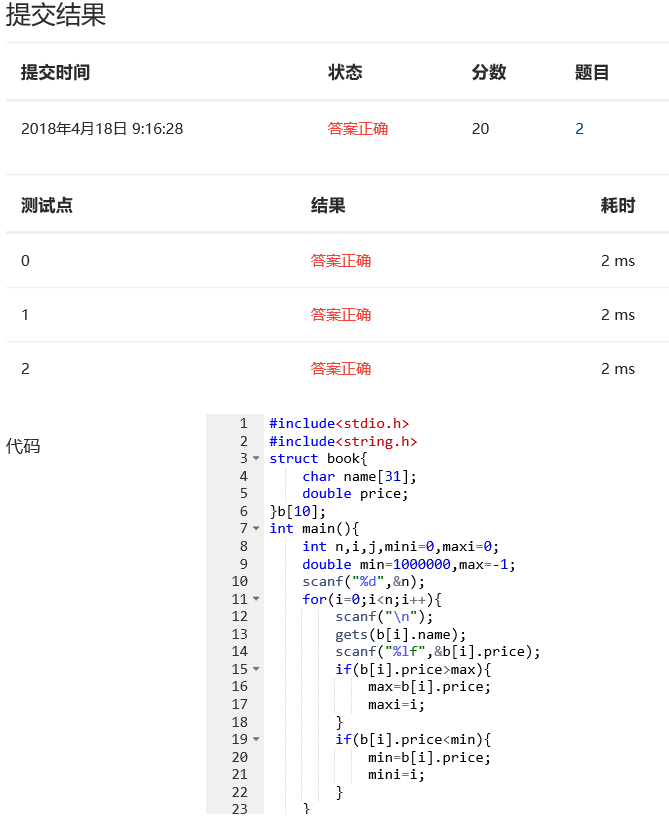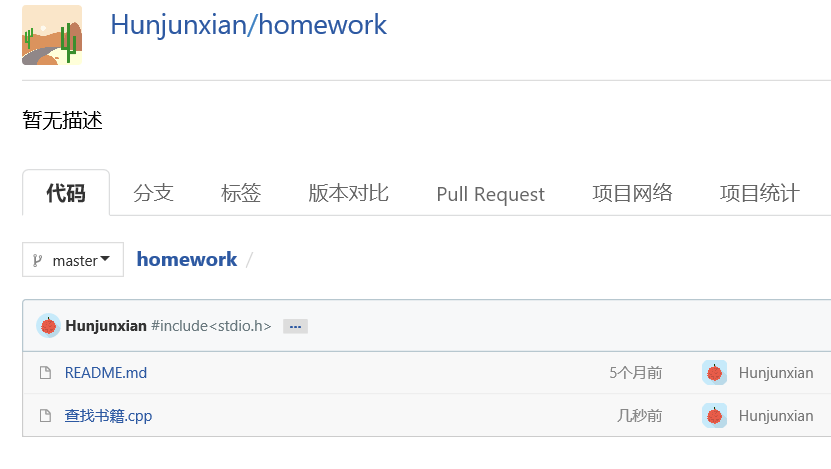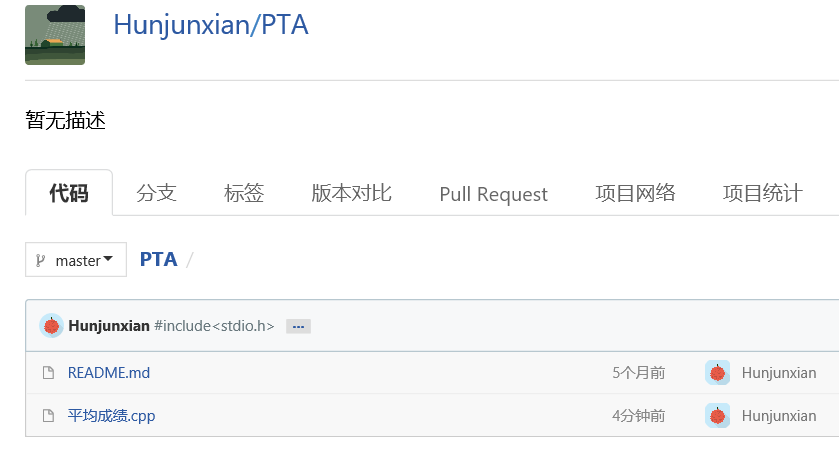1.最普通的int型指针:

int a=5;

int*p= &a;

p=&a;

2.用一个初始化了的指针可以对新定义的指针进行初始化:

int a;

int*p=&a;

int*q=p;

3.指针的运算

int a=4;

int *p=&a;

int b=*p++;

* 与++优先级相同

p++等效与p+sizeof(int)

p+5等效于p+5×sizeof(int)

4.指针在函数的调用

void swap(int*p_one,int *p_two){

int *temp

temp=p_one;

p_one=p_two;

p_two=temp;

}

void swap(int*p_one,int *p_two){

int *temp;

*temp=*p_one;

*p_one=*p_two;

*p_two=*temp;

}

5.数组与指针

int a={1,2,3,4,5}

(1)定义一个指向数组元素的指针变量

int a={1,2,3,4,5};

int*p=a;

(2)利用指针访问数组的几种方式

int a[i]

1.*(a+i)

2.*(p+i)

3.p[i]

学习进度：posted @ 2018-04-18 09:02  句号？  阅读(160)  评论(0编辑  收藏  举报